Friday, September 29, 2023
HomeAIMachine LearningIntroducing all the machine learning libraries! | 5 recommended libraries for beginners

# Introducing all the machine learning libraries! | 5 recommended libraries for beginners

A ” library ” in programming is a program that allows anyone to easily use a series of highly versatile processes.

By using the library according to your purpose, you can easily design and execute the program without creating the program from scratch.

Especially in machine learning, which has a complicated process, various libraries have been developed and are widely used.

In this article, we will introduce in detail the types of libraries in machine learning, while taking up the library of Python, which is a typical programming language in the field of machine learning.

• What is a Python library
• Pretreatment stage
• train the model
• Libraries for preprocessing
• Numpy
• Scipy
• pandas
• Matplotlib
• Seaborn
• scikit-learn
• Image processing
• natural language processing
• gensim
• Libraries for model training
• machine learning
• scikit-learn
• deep learning
• TensorFlow
• Pytorch
• reinforcement learning
• 5 Recommended Machine Learning Libraries That You Want to Learn First
• summary

## What is a Python library

Python is a programming language famous for its rich library. Although this article will only introduce libraries related to machine learning, Python has a wide range of libraries.

By using such a rich library, Python can be used in a wide range of applications such as numerical calculation, data analysis, game and application development.

In this article, the machine learning process is roughly divided into “preprocessing” and “model learning”, and we will explain the libraries that are often used in that process.

### Pretreatment stage

Data processing is the process of examining and shaping the data before analyzing it using AI.

The process is a bit far from the image of “creating AI”, but AI is an analysis tool, so data processing prior to analysis is done by hand.

Therefore, data processing is where the skills and individuality of AI developers come into play, and a considerable amount of time is devoted to this process.

### train the model

Next is the process of learning the data. The methods can be broadly divided into three categories: supervised learning, unsupervised learning , and reinforcement learning .

In addition, a method that uses a mechanism called a neural network in a multi-layered structure is called ” deep learning.”

If the accuracy of the results is poor, the preprocessing of the data may be redone depending on the situation.

## Libraries for preprocessing

Preprocessing involves cleaning, concatenating, and transforming data to make it suitable for analysis, capturing its inherent properties and possibly manipulating variables.

The exact process depends on your data and purpose. Therefore, it may be necessary to detect outliers or compute statistical features for numerical data.

It is also important to visualize the data in graphs to make it easier to objectively grasp the characteristics of the data. In addition, specific preprocessing is required especially for image/audio processing and natural language processing.

### Numpy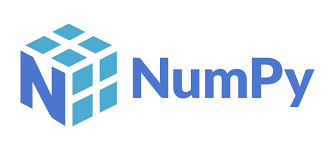Numpy is a library for numerical computation, and is good at numerical processing such as operations on vectors and matrices.

The contents of Numpy are implemented in C language (or a language closer to machine language than Fortnan), so even large amounts of data can be processed in a short time.

Python also implements the concept of representing multidimensional arrays called “lists” as a default function, but complex calculations require complicated processing internally.

Numpy, on the other hand, can handle such complex calculations quickly and easily.

### ScipyScipy is another representative numerical calculation library. Compared to Numpy, it has a huge structure and is divided into subpackages according to functions and uses.

So you can do more with Scipy than you can with Numpy.

### pandas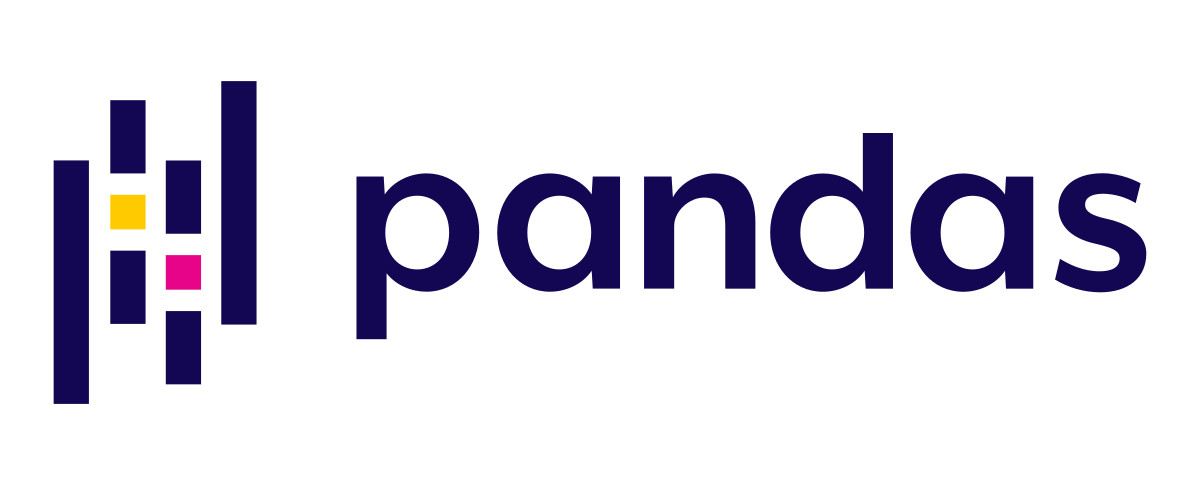Pandas is suitable for concatenating and splitting data, capturing statistical features such as the mean and standard deviation of data, and is a library that is often used in the cleaning stage such as processing outliers.

Also, Pandas can display table data in a nice way.

### Matplotlib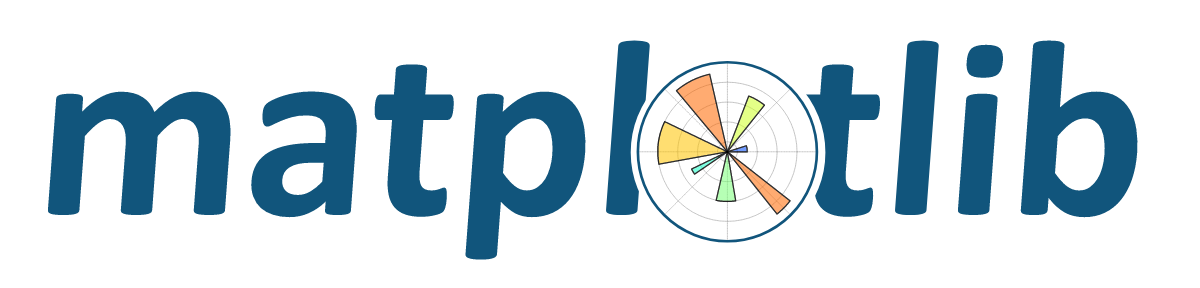Matplotlib is a graph drawing library. By drawing complex data on a graph, you will be able to understand trends, deviations, and characteristics. The full range of graph types and display customization functions is a masterpiece.

Also, by using Matplotlib, you can create easy-to-read graphs. It is possible to customize not only the shape of the graph, but also the colors and characters.

### Seaborn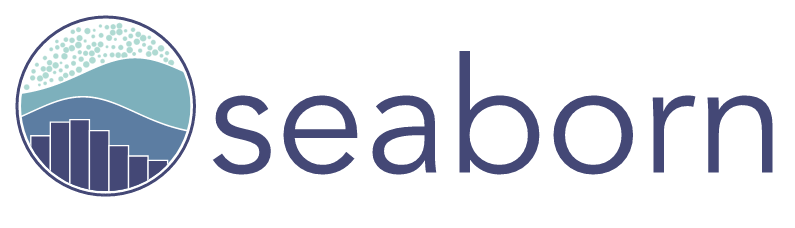Seaborn is a leading data visualization library, functionally similar to Matplotlib. Create sophisticated graphs with less code.

### scikit-learn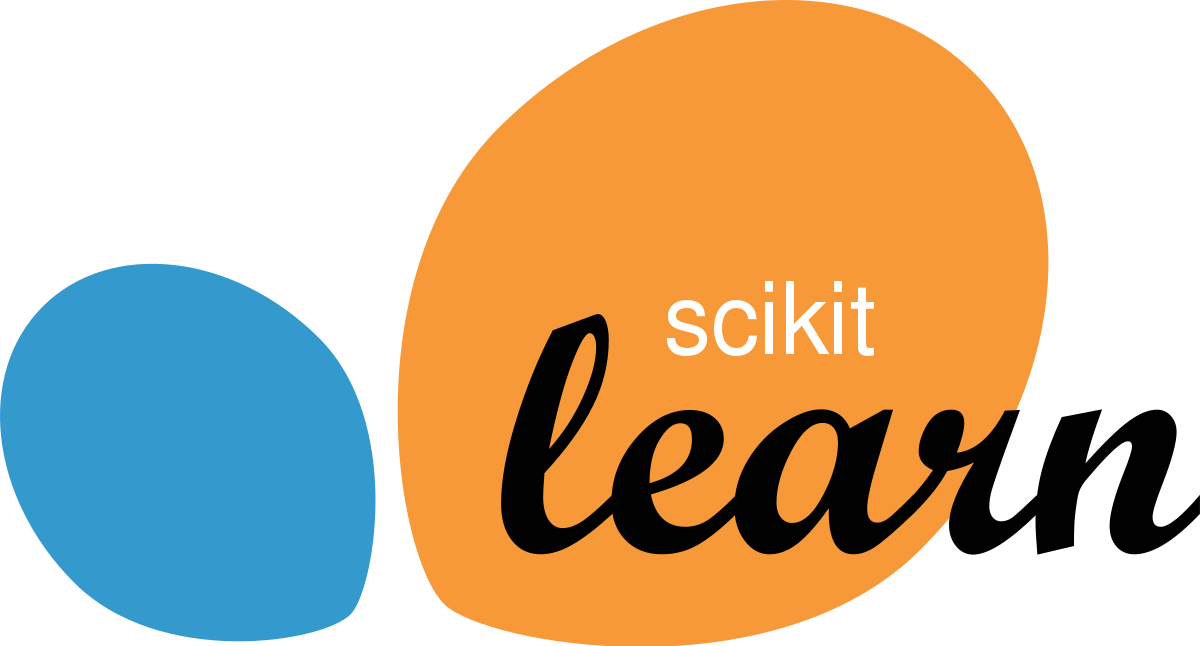A detailed explanation of scikit-learn will be given in the model training section below, but here we will introduce the case where scikit-learn is used in the preprocessing stage.

Scikit-learn has many functions that perform complex preprocessing all at once, making it possible to easily perform technical processing such as standardization and regularization of data.

### Image processing

OpenCV and pillow are useful libraries for image processing. OpenCV developed by Intel is a traditional open source library in the field of image processing.

In addition to file conversion and deformation, it is equipped with a wealth of useful functions for image processing using AI, such as object recognition and face recognition.

### natural language processing

Natural language processing is a technology that allows computers to handle natural language, that is, words that we use every day.

Computers are designed and operated using formal languages ​​(concepts that are paired with natural languages, such as programming languages). “Relationships” can be processed.

### gensim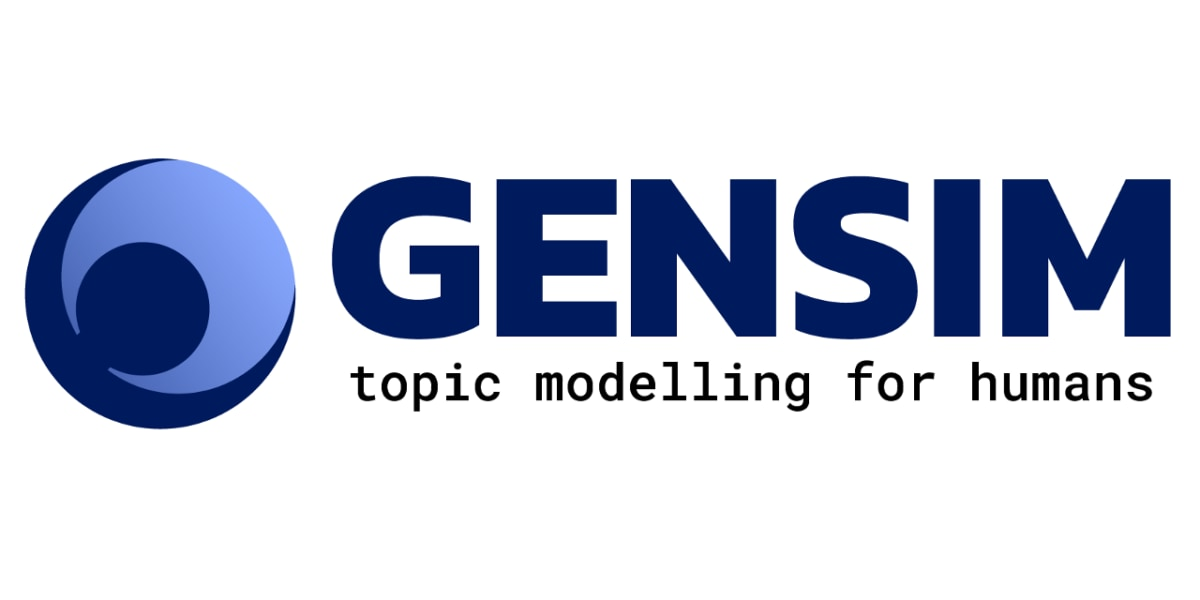gensim is a library that implements a technology for natural language processing called “topic model”.

“Topic” is often translated as “subject” or “topic” in the field of linguistics. A topic model can determine which topic a document belongs to, and can handle words that occur frequently in each topic.

Gensim is often used with a model for natural language processing called word2vec. word2vec is an epoch-making technology that enables mathematical processing of meanings and relationships in natural language by introducing the concept of “semantic vectors”.

## Libraries for model training

So far, I have introduced libraries that are mainly active in the preprocessing stage. Next, let’s take a look at libraries that are often used when actually learning a model.

In this article, we will introduce two of the model learning techniques, reinforcement learning and deep learning, which require slightly special techniques, separately from basic machine learning.

### machine learning

Machine learning libraries implement various machine learning algorithms. Different algorithms are used depending on the purpose of analysis and the characteristics of the data.

### scikit-learnscikit-learn is probably the most famous machine learning library. Most machine learning methods are in this library.

It also implements the tools necessary for the entire machine learning process, such as the “evaluation index for learning results of techniques and models” that randomly splits training data and test data.

Another feature is that it is compatible with Numpy and Scipy, which are numerical calculation libraries for python. Since it is compatible with multiple other libraries, using scikit-learn makes it possible to design and operate machine learning more intuitively.

scikit-learn also focuses on learning materials, and the official tutorial is evaluated as a machine learning material for beginners.

### deep learning

Among machine learning methods, deep learning is a method that uses neural networks, which are models that mimic neural circuits, overlaid in multiple layers.

There are two typical deep learning specialized libraries: TensorFlow developed by Google and Pytorch developed by Facebook.

### TensorFlow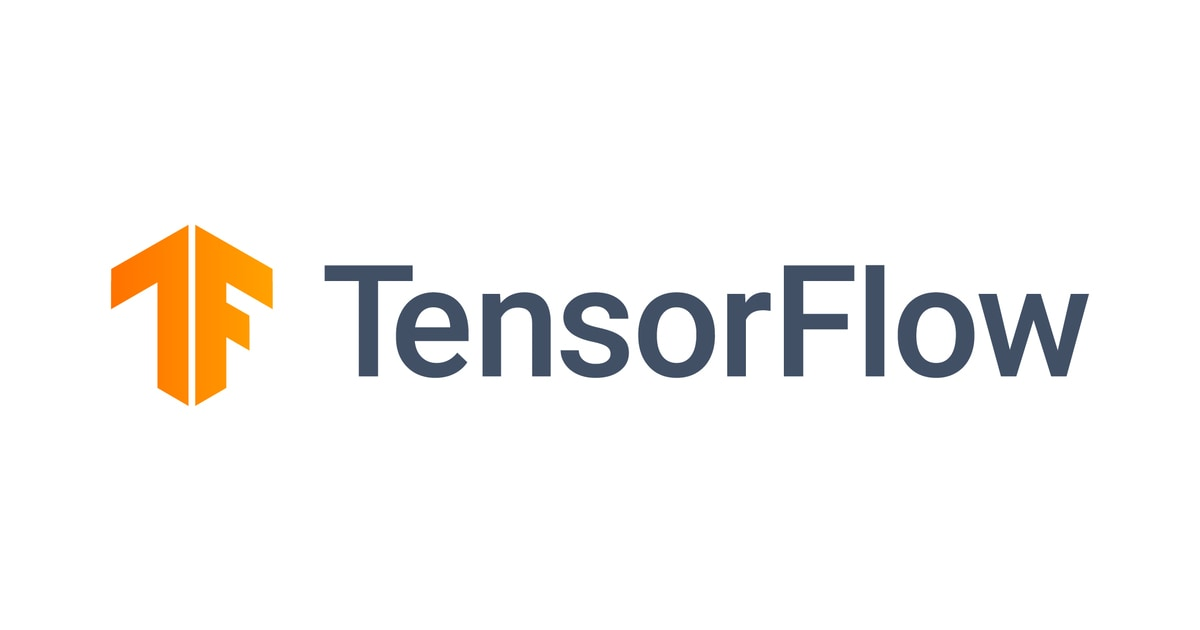TensorFlow is an open source deep learning framework released in 2015, and the official version was released in 2017. It supports major OSs and multiple programming languages, and its strength is its high versatility.

In addition, TensorFlow is characterized by a large number of users, and there are abundant learning materials such as books and articles on the Internet. Therefore, it is a recommended library for those who want to touch deep learning for the first time.

### Pytorch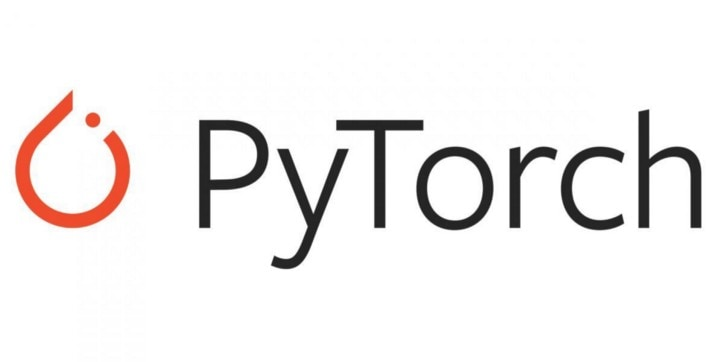Pytorch is an open source deep learning framework released in 2016. Although it is inferior to TensorFlow in terms of general penetration, it has a high reputation for intuitive and easy-to-understand code creation and model manipulation, and it is gaining popularity mainly in the field of research.

### reinforcement learning

We will introduce Dopamine and PFRL as examples of libraries specialized in ” reinforcement learning ” used in autonomous driving and game AI .

Dopamine is a TensorFlow-based reinforcement learning framework that implements multiple reinforcement learning algorithms around DQN (Deep Q-Network).

In addition, PFRL (formerly ChainerRL) can perform so-called deep reinforcement learning using multi-layered neural networks on Pytorch.

## 5 Recommended Machine Learning Libraries That You Want to Learn First

So far, we have provided a comprehensive introduction to the major machine learning libraries. From here, I will introduce what library should be studied first by those who have never used a Python library for machine learning, and how to learn it.

As a preprocessing step, it’s good to learn three sets: Numpy, Pandas, and Matplotlib. These three are also treated as a set in major commentary books.

However, Scipy is sometimes cited as an alternative to Numpy when it comes to math library recommendations.

It is true that Scipy has more functions than Numpy, but on the contrary, Numpy has the advantage of being simple to operate, so it is easy to understand if you study Numpy, especially for those who are new to numerical calculation libraries.

It is recommended to learn scikit-learn for machine learning library and TensorFlow for deep learning library first.

These two are used by so many people in the wild that there is a wealth of information and learning material associated with the libraries. In addition, it also has the advantage of being compatible with popular development environments for machine learning such as Jupyter Notebook and Google Colaboratory.

#### Jupyter Notebook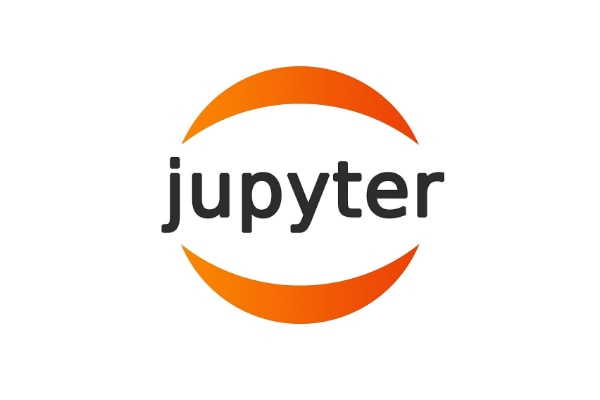Jupyter Notebook is a popular environment, especially in the field of data analysis. It is included as a package in the Anaconda platform.

The biggest feature is that you can run the program line by line and edit the code while checking the operation. As such, many books and educational materials recommend using Jupyter Notebooks.Google Colaboratory is a development environment often used in the field of deep learning.

Deep learning, which deals with huge data groups called big data, requires enormous computational processing to execute. However, in most cases, it cannot be processed with the power of general-purpose personal computers.

On the other hand, Google Colaboratory can access Google’s GPU (processor with higher computing power) for free, so anyone can easily execute deep learning programs.

Also, since Google Colaboratory is based on Jupyter Notebook, the operational feeling is almost the same and it is easy to use.

## summary

In this article, we have introduced libraries that are often used in machine learning.

All Python libraries are characterized by intuitive and easy-to-understand operations.

Especially for those who want to try machine learning for the first time, it is recommended to touch on various libraries after learning the basic syntax of Python.

Of course, Python’s libraries are not limited to the machine learning domain. One of the big reasons why I recommend Python libraries is that they can be combined with libraries in various fields such as machine learning and web application development.

RELATED ARTICLES

Recommended